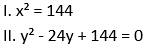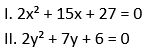Dear Aspirants,

### Quantitative Aptitude Quiz For IBPS PO/Clerk Prelims

Numerical Ability or Quantitative Aptitude Section has given heebie-jeebies to the aspirants when they appear for a banking examination. As the level of every other section is only getting complex and convoluted, there is no doubt that this section, too, makes your blood run cold. The questions asked in this section are calculative and very time-consuming. But once dealt with proper strategy, speed, and accuracy, this section can get you the maximum marks in the examination. Following is the Quantitative Aptitude quiz to help you practice with the best of latest pattern questions.
WATCH VIDEO SOLUTION HERE

Directions (1-5): Find the approximate value of the following questions.

Q1. 324.995 × 15.98 ÷ 4.002 + 36.88 = ?
1340
1230
1540
1380
1390

Q2. 1164 × 128 ÷ 8.008 + 969.007 = ?
18800
19393
19593
19200
20293

Q3.58
56
61
52
62

Q4. 69.008% of 699.98 + 32.99% of 399.999 = ?
615
645
675
715
815

Q5. (9321 + 5406 + 1001) ÷ (498 + 929 + 660) = ?
13.5
4.5
2.5
7.5
21.5

Directions (6-10): What will come in place of question mark (?) in the following number series?

Q6. 148, 152, 161, 177, ? , 238
208
214
202
198
192

Q7. 339, 355, 323, 371, 307, ?
407
354
328
387
388

Q8. 5, 14, 40, 117, 347, ?
920
745
1124
1036
694

Q9. 12, 24, 96, 576, ? , 46080
3542
3890
4248
4608
3246

Q10. 156, 468, 780, ? , 1404, 1716
1096
1092
1290
9610
1910

Directions (11-15): In the following questions two equations numbered I and II are given. You have to solve both the equations and give answer

Q11.If x < y
If x ≤ y
If y < x
If y ≤ x
If x =y or if no relationship can be established.

Q12.If x < y
If x ≤ y
If y < x
If y ≤ x
If x =y or if no relationship can be established.

Q13.If x < y
If x ≤ y
If y < x
If y ≤ x
If x =y or if no relationship can be established.

Q14.If x < y
If x ≤ y
If y < x
If y ≤ x
If x =y or if no relationship can be established.

Q15.If x < y
If x ≤ y
If y < x
If y ≤ x
If x =y or if no relationship can be established.

You May also like to Read:

Test where you stand in the Competition with Online Test Series
to get 25% Off Today

Get Classroom at Your Fingertips with Video Courses

Enroll in LIVE BATCHES

Get Latest Printed Edition BOOKS

Study Anytime with eBOOKS